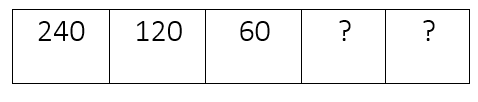Mathematics
Easy

Question

# A division rule was used to make the pattern of numbers in the table.If the pattern continues, then the next two numbers will be

## 30, 15    30, 10    20, 14    20, 10Hint:

## The correct answer is: 30, 15

### The given sequence is 240, 120, 60We have to find the next two terms in this sequence.Let’s find the relation between the first and second term. It will be the constant for all the terms.Factor = first term ÷ second term= 240 ÷ 120= 2So, the factor by which we are dividing each term is 2.Let’s see how it works for every term.240 ÷ 2 = 120120 ÷ 2 = 60Now, let’s find the next terms.The last term from the above sequence is 60So, we will divide the term by 2.60 ÷ 2 = 30This will be our new next term. We will take this term and divide by 2.30 ÷ 2 = 15We got the two new terms, “30” and “15”. We will see which option matches these values.The complete sequence will be 240, 120, 60, 30, 15And the option which is ’30, 15’, is the required option.

For such questions, we should know the basic operations. When the rule used to write the pattern is not mentioned, we have to use all four operations to check the pattern. The operations are addition, subtraction, division, and multiplication.

### Related Questions to study#### With Turito Foundation.#### Get an Expert Advice From Turito.